Next: Standard prefixes Up: Introduction Previous: What is classical mechanics?

mks units

The first principle of any exact science is measurement. In mechanics there are three fundamental quantities which are subject to measurement:
1. Intervals in space: i.e., lengths.
2. Quantities of inertia, or mass, possessed by various bodies.
3. Intervals in time.
Any other type of measurement in mechanics can be reduced to some combination of measurements of these three quantities.

Each of the three fundamental quantities--length, mass, and time--is measured with respect to some convenient standard. The system of units currently used by all scientists, and most engineers, is called the mks system--after the first initials of the names of the units of length, mass, and time, respectively, in this system: i.e., the meter, the kilogram, and the second.

The mks unit of length is the meter (symbol m), which was formerly the distance between two scratches on a platinum-iridium alloy bar kept at the International Bureau of Metric Standard in Sèvres, France, but is now defined as the distance occupied by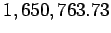wavelengths of light of the orange-red spectral line of the isotope Krypton 86 in vacuum.

The mks unit of mass is the kilogram (symbol kg), which is defined as the mass of a platinum-iridium alloy cylinder kept at the International Bureau of Metric Standard in Sèvres, France.

The mks unit of time is the second (symbol s), which was formerly defined in terms of the Earth's rotation, but is now defined as the time for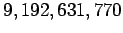oscillations associated with the transition between the two hyperfine levels of the ground state of the isotope Cesium 133.

In addition to the three fundamental quantities, classical mechanics also deals with derived quantities, such as velocity, acceleration, momentum, angular momentum, etc. Each of these derived quantities can be reduced to some particular combination of length, mass, and time. The mks units of these derived quantities are, therefore, the corresponding combinations of the mks units of length, mass, and time. For instance, a velocity can be reduced to a length divided by a time. Hence, the mks units of velocity are meters per second:(1)

Here,stands for a velocity,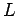for a length, and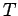for a time, whereas the operator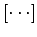represents the units, or dimensions, of the quantity contained within the brackets. Momentum can be reduced to a mass times a velocity. Hence, the mks units of momentum are kilogram-meters per second: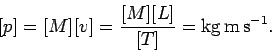(2)

Here,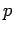stands for a momentum, and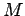for a mass. In this manner, the mks units of all derived quantities appearing in classical dynamics can easily be obtained.Next: Standard prefixes Up: Introduction Previous: What is classical mechanics?
Richard Fitzpatrick 2006-02-02